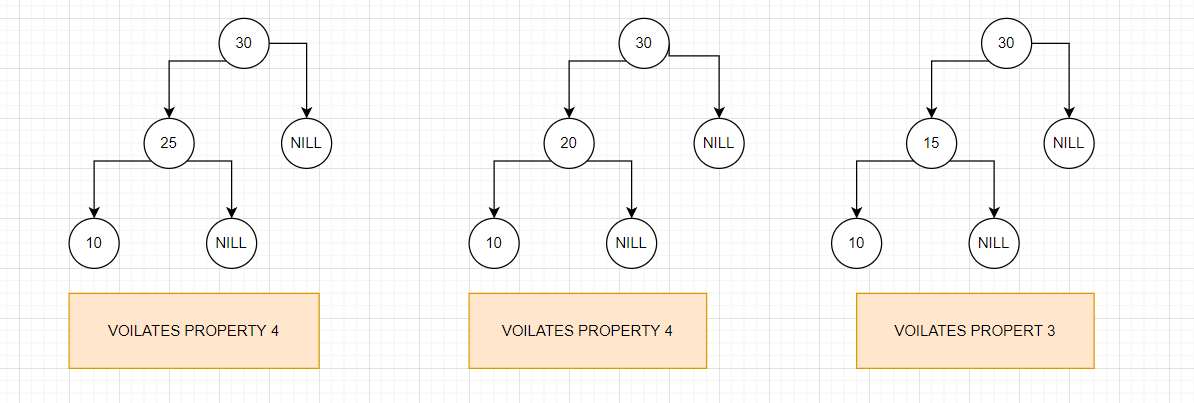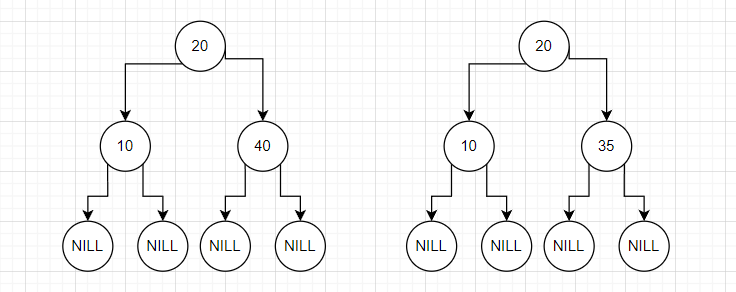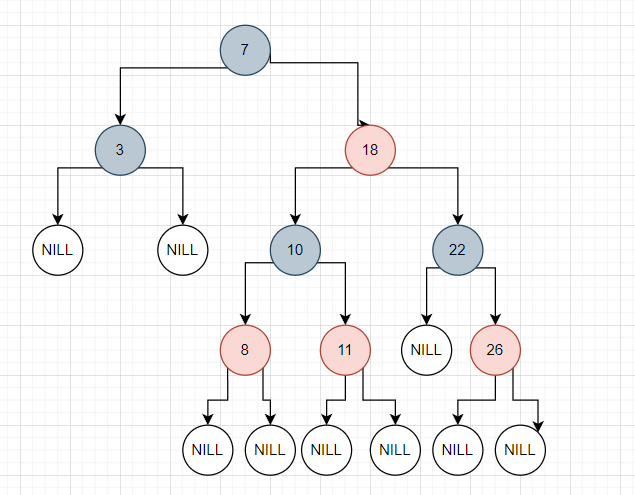# Introduction To Red-Black Trees

## Introduction:

A red-black tree is a form of self-balancing binary seek tree in which each node has a further bit, and that bit is regularly interpreted because of the colouration (crimson or black). Those colours are used to make sure that the tree remains balanced, all through insertions and deletions. Even though the balance of the tree isn't always best, it is ideal to reduce the looking time and hold it round o(log n) time, where n is the total quantity of factors in the tree. This tree was invented in 1972 with the aid of Rudolf Bayer.

As each node requires the handiest one little bit of area to store the colouration records, these kinds of bushes show an equal reminiscence footprint to the conventional (uncoloured) binary seek tree.

## Rules for Red-Black Tree:

1. A node can have Either Red colour or Black Colour.
2. Root nodes always have Black Colour.
3. No two Red nodes can be adjacent to each other.
4. The path from any Node to any descendants null node has the same count of black nodes.

## Why do we Use Red Black Trees?

Most of the best operations (e.G.,  max, min, insert, delete.. Etc) take o(h) time in which (h) is the height of the Bst. The cost of these operations may also emerge as o(n) for a skewed binary tree. If we are sure that the height of the binary tree stays o(log n) after each insertion and deletion, then we can guarantee an upper certain of o(log n) for all these operations. The height of a red-black tree is constantly o(log n), wherein n is the number of nodes inside the tree.

Here “n” represents the total no of the element that is present in the red Black Tree.

## Comparision B/w Red Black tree and AVL tree:

The AVL tree is more balanced than the Red-black tree, but when the deletion of insertion operation is performed, the AVL tree requires more number of rotations. So when an operation requires frequent insertion and deletion then a Red-black tree is preferred more than the AVL tree to reduce time complexity.

#### How does a Red-Black Tree ensure balance?The following is not a Red-Black tree because the chain of three-nodes is not possible.## The following is a red-black tree with three keys.

1. The black height of the tree is the total count of black nodes on a path from the base node to a leaf node. Leaf nodes also are counted as black nodes. So, the red-black tree of height (h) has the black height >= h/2.
2. The height of a red-black tree with n nodes is h<= 2 log2(n + 1)
3. All bottom leaf nodes are black.
4. The red-Black tree is the remarkable variety of the Binary Tree.
5. No of Black Nodes is defined as the no of black nodes from the nodes to the given node that was no of an ancestor.

## Black Height of Red-Black Tree:

The count of nodes from a node to its farthest descendant leaf is not quite twice because of the number of nodes to the closest descendant leaf.

Height h of red-black Tree is >=h/2;

Note: Red-Black Tree which has a height h and nodes count(n) has height<= 2Log2(n+1);

## Searching in Red Black Tree:

Since the red-black tree is a special case of the binary tree, searching is similar to binary tree searching.

Algorithm:

For more refer coding ninjas tree article.

ExampleSearch 11 in the given Tree

Solutions:

1. Start from the root.
2. Compare the element with the root element if root value> our value then recurse the left else if it is less than recuse the right half.
3. If the root value is equal to our value then return true.

## Application:

1. It is used in CPU scheduling in an operating system like Linux.
2. Mysql also uses the Red-Black Tree for storing the indexes of tables.
3. They are also used in the k-means clustering algorithm for reducing complexity.

### 1:- What is Red-Black Tree?

-> Red-Black Tree is a variety of Binary Trees in which the last node of the Tree is Null.

### 2:- Why do we use the Red-black Tree over the AVL tree?

-> In every case, the time complexity of the Red-black Tree is Less than the AVL tree.

### 3:- What is the Time complexity of the Red-Black Tree for insertion, deletion and searching?

-> Time complexity for every case is o(log(n));

### 4:- How searching takes place in Red-Black Tree?

-> Searching in the red-black Tree is similar to searching in the AVL tree.

### 5:- What are the applications of the Red-Black Tree?

-> It is mainly used in Linux OS and MySql software.

## Key Takeaways:

This article covered the introduction of red-black, and side by side we have covered a comparison between red-black tree and  AVL trees.